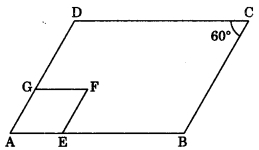# CBSEtips.in

## Saturday, 20 February 2021

### CBSE Class 9 Maths - MCQ and Online Tests - Unit 8 - Quadrilaterals

#### CBSE Class 9 Maths – MCQ and Online Tests – Unit 8 – Quadrilaterals

Every year CBSE  schools conducts Annual Assessment exams for 6,7,8,9,11th standards. These exams are very competitive to all the students. So our website provides online tests for all the 6,7,8,9,11th standard’s subjects. These tests are also very effective and useful for those who preparing for any competitive exams like Olympiad etc. It can boost their preparation level and confidence level by attempting these chapter wise online tests.

These online tests are based on latest CBSE syllabus. While attempting these our students can identify the weak lessons and continuously practice those lessons for attaining high marks. It also helps to revise the NCERT textbooks thoroughly.

#### CBSE Class 9 Maths – MCQ and Online Tests – Unit 8 – Quadrilaterals

Question 1.
If one angle of a parallelogram is 24° less than twice the smallest angle, then the measure of the largest angle of a parallelogram is
(a) 176°
(b) 68°
(c) 112°
(d) 102°

Question 2.
If angles A, B, C and D of a quadrilateral ABCD, taken in order, are in the ratio 3 : 7 : 6 : 4, then ABCD is a
(a) rhombus
(b) parallelogram
(c) trapezium
(d) kite.

Question 3.
If the diagonal of a rhombus are 18 cm and 24 cm respectively, then its side is equal to
(a) 16 cm
(b) 15 cm
(c) 20 cm
(d) 17 cm

Answer: (b) 15 cm

Question 4.
In the given figure, ABCD is a parallelogram. Find the value of x.(a) 25°
(b) 60°
(c) 75°
(d) 45°

Question 5.
The diagonals AC and BD of a || gm ABCD intersect each other at the point O. If ?DAC = 32° and ?AOB = 70°, then ?DBC is equal to
(a) 24°
(b) 86°
(c) 38°
(d) 32°

Question 6.
ABCD is a rhombus such that ?ABC = 40°, then ?ADC is equal to
(a) 40°
(b) 45°
(c) 50°
(d) 20°

Question 7.
In the following figure, ABCD and AEFG are two parallelograms. If ?C = 60°, then ?GFE is(a) 30°
(b) 60°
(c) 90°
(d) 120°

Question 8.
The bisectors of any two adjacent angles of a || gm intersect at
(a) 30°
(b) 45°
(c) 60°
(d) 90°

Question 9.
A diagonal of a rectangle is inclined to one side of the rectangle at 25°. The acute angle between the diagonals is
(a) 55°
(b) 50°
(c) 40°
(d) 25°

Question 10.
ABCD is a rhombus such that ?ACB = 40°, then ?ADB is
(a) 40°
(b) 45°
(c) 50°
(d) 60°